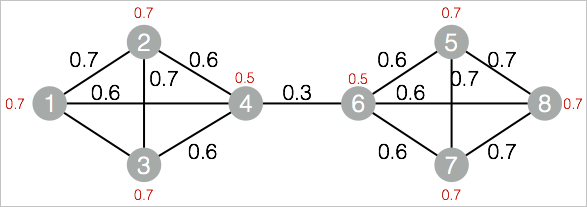A label propagation algorithm (LPA) is a semi-supervised machine learning algorithm. The labels of a node (community) depend on those of the neighboring nodes. The degree of dependence is determined by the similarity between nodes. Data becomes stable by iterative propagation updates. This topic describes the Label Propagation Clustering component provided by Machine Learning Studio.

## Background information

Graph clustering is used to divide a graph into subgraphs based on the topology of the graph. Therefore, the links between the nodes in a subgraph are more than the links between the subgraphs.

You can configure the component by using one of the following methods:

## Machine Learning Platform for AI console

Tab Parameter Description
Fields Settings Vertex Table: Vertex Column The vertex column in the vertex table.
Vertex Table: Weight Column The vertex weight column in the vertex table.
Edge Table: Source Vertex Column The start vertex column in the edge table.
Edge Table: Target Vertex Column The end vertex column in the edge table.
Edge Table: Weight Column The edge weight column in the edge table.
Parameters Settings Maximum Iterations The default value is 30. This parameter is optional.
Tuning Workers The number of vertices for parallel job execution. The parallelism level and framework communication costs increase with the value of this parameter.
Memory Size Per Worker (MB) The maximum size of memory that a single job can use. By default, the system allocates 4,096 MB for each job. If the used memory size exceeds the value of this parameter, the OutOfMemory exception is reported.

## PAI command

``````PAI -name LabelPropagationClustering
-project algo_public
-DinputEdgeTableName=LabelPropagationClustering_func_test_edge
-DfromVertexCol=flow_out_id
-DtoVertexCol=flow_in_id
-DinputVertexTableName=LabelPropagationClustering_func_test_node
-DvertexCol=node
-DoutputTableName=LabelPropagationClustering_func_test_result
-DhasEdgeWeight=true
-DedgeWeightCol=edge_weight
-DhasVertexWeight=true
-DvertexWeightCol=node_weight
-DrandSelect=true
-DmaxIter=100;``````
Parameter Required Description Default value
inputEdgeTableName Yes The name of the input edge table. No default value
inputEdgeTablePartitions No The partitions in the input edge table. Full table
fromVertexCol Yes The start vertex column in the input edge table. No default value
toVertexCol Yes The end vertex column in the input edge table. No default value
inputVertexTableName Yes The name of the input vertex table. No default value
inputVertexTablePartitions No The partitions in the input vertex table. Full table
vertexCol Yes The vertex column in the input vertex table. No default value
outputTableName Yes The name of the output table. No default value
outputTablePartitions No The partitions in the output table. No default value
lifecycle No The lifecycle of the output table. No default value
workerNum No The number of vertices for parallel job execution. The parallelism level and framework communication costs increase with the value of this parameter. Not configured
workerMem No The maximum size of memory that a single job can use. By default, the system allocates 4,096 MB for each job. If the used memory size exceeds the value of this parameter, the OutOfMemory exception is reported. 4096
splitSize No The data split size. 64
hasEdgeWeight No Specifies whether the edges in the input edge table have weights. false
edgeWeightCol No The edge weight column in the input edge table. No default value
hasVertexWeight No Specifies whether the vertices in the input vertex table have weights. false
vertexWeightCol No The vertex weight column in the input vertex table. No default value
randSelect No Specifies whether the maximum label value is to be randomly selected. false
maxIter No The maximum number of iterations. 30

## Examples

1. Generate training data.
``````drop table if exists LabelPropagationClustering_func_test_edge;
create table LabelPropagationClustering_func_test_edge as
select * from
(
select '1' as flow_out_id,'2' as flow_in_id,0.7 as edge_weight from dual
union all
select '1' as flow_out_id,'3' as flow_in_id,0.7 as edge_weight from dual
union all
select '1' as flow_out_id,'4' as flow_in_id,0.6 as edge_weight from dual
union all
select '2' as flow_out_id,'3' as flow_in_id,0.7 as edge_weight from dual
union all
select '2' as flow_out_id,'4' as flow_in_id,0.6 as edge_weight from dual
union all
select '3' as flow_out_id,'4' as flow_in_id,0.6 as edge_weight from dual
union all
select '4' as flow_out_id,'6' as flow_in_id,0.3 as edge_weight from dual
union all
select '5' as flow_out_id,'6' as flow_in_id,0.6 as edge_weight from dual
union all
select '5' as flow_out_id,'7' as flow_in_id,0.7 as edge_weight from dual
union all
select '5' as flow_out_id,'8' as flow_in_id,0.7 as edge_weight from dual
union all
select '6' as flow_out_id,'7' as flow_in_id,0.6 as edge_weight from dual
union all
select '6' as flow_out_id,'8' as flow_in_id,0.6 as edge_weight from dual
union all
select '7' as flow_out_id,'8' as flow_in_id,0.7 as edge_weight from dual
)tmp
;
drop table if exists LabelPropagationClustering_func_test_node;
create table LabelPropagationClustering_func_test_node as
select * from
(
select '1' as node,0.7 as node_weight from dual
union all
select '2' as node,0.7 as node_weight from dual
union all
select '3' as node,0.7 as node_weight from dual
union all
select '4' as node,0.5 as node_weight from dual
union all
select '5' as node,0.7 as node_weight from dual
union all
select '6' as node,0.5 as node_weight from dual
union all
select '7' as node,0.7 as node_weight from dual
union all
select '8' as node,0.7 as node_weight from dual
)tmp;``````
The following figure shows the structure of the label propagation clustering graph.2. View training results.
``````+------+------------+
| node | group_id   |
+------+------------+
| 1    | 1          |
| 2    | 1          |
| 3    | 1          |
| 4    | 1          |
| 5    | 5          |
| 6    | 5          |
| 7    | 5          |
| 8    | 5          |
+------+------------+``````